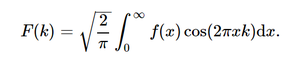# sympy.integrals.transforms.cosine_transform() in python

With the help of cosine_transform() method, we can compute the cosine transformation and return the transformed function by using this method.cosine transformation

Syntax : cosine_transform(f, x, k, **hints)

Return : Return the transformed function.

Example #1 :

In this example we can see that by using cosine_transform() method, we are able to compute the cosine transformation and return the transformed function.

## Python3

 `# import cosine_transform ` `from` `sympy ``import` `cosine_transform, exp, sqrt, cos ` `from` `sympy.abc ``import` `x, k, a ` ` `  `# Using cosine_transform() method ` `gfg ``=` `cosine_transform(exp(``-``a ``*` `x), x, k) ` ` `  `print``(gfg)`

Output :

sqrt(2)*a/(sqrt(pi)*(a**2 + k**2))

Example #2 :

## Python3

 `# import cosine_transform ` `from` `sympy ``import` `cosine_transform, exp, sqrt, cos ` `from` `sympy.abc ``import` `x, k, a ` ` `  `# Using cosine_transform() method ` `gfg ``=` `cosine_transform(exp(``-``a ``*` `x), x, ``5``) ` ` `  `print``(gfg)`

Output :

sqrt(2)*a/(sqrt(pi)*(a**2 + 25))

My Personal Notes arrow_drop_upCheck out this Author's contributed articles.

If you like GeeksforGeeks and would like to contribute, you can also write an article using contribute.geeksforgeeks.org or mail your article to contribute@geeksforgeeks.org. See your article appearing on the GeeksforGeeks main page and help other Geeks.

Please Improve this article if you find anything incorrect by clicking on the "Improve Article" button below.

Article Tags :

Be the First to upvote.

Please write to us at contribute@geeksforgeeks.org to report any issue with the above content.